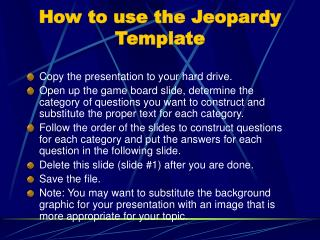Download PresentationHow to use the Jeopardy Template

# How to use the Jeopardy Template

Télécharger la présentation## How to use the Jeopardy Template

- - - - - - - - - - - - - - - - - - - - - - - - - - - E N D - - - - - - - - - - - - - - - - - - - - - - - - - - -
##### Presentation Transcript

1. How to use the Jeopardy Template • Copy the presentation to your hard drive. • Open up the game board slide, determine the category of questions you want to construct and substitute the proper text for each category. • Follow the order of the slides to construct questions for each category and put the answers for each question in the following slide. • Delete this slide (slide #1) after you are done. • Save the file. • Note: You may want to substitute the background graphic for your presentation with an image that is more appropriate for your topic.

2. LET’S PLAY JEOPARDY!!

3. Jeopardy Basic Operations Expressions & Equations Decimals Properties Place Value Q \$100 Q \$100 Q \$100 Q \$100 Q \$100 Q \$200 Q \$200 Q \$200 Q \$200 Q \$200 Q \$300 Q \$300 Q \$300 Q \$300 Q \$300 Q \$400 Q \$400 Q \$400 Q \$400 Q \$400 Q \$500 Q \$500 Q \$500 Q \$500 Q \$500 Final Jeopardy

4. \$100 Question from Basic Operations • True or False? • You use ADDITION to find the SUM.

5. \$200 Question from Basic Operations • Find the SUM: • 208 + 816 + 7,049

6. \$300 Question from Basic Operations • Find the DIFFERENCE: • 17,001 – 8,542

7. \$400 Question from Basic Operations DOUBLE JEOPARDY • Find the PRODUCT: • 119 x 54

8. \$500 Question from Basic Operations • Solve: • Ali, Julia and Jorge went out for ice-cream. Ali ordered 2 scoops. Julia and Jorge each ordered 3 scoops. Each scoop cost \$1.25. How much more money did Julia pay than Ali?

9. \$500 Answer from Basic Operations Julia spent \$1.25 more than Ali. (So did Jorge!)

10. \$100 Question from Expressions & Equations • True or False? • An EXPRESSION is a mathematical sentence WITH = sign.

11. \$100 Answer from Expressions & Equations • False • An EQUATION has an = sign • An EXPRESSION does not

12. \$200 Question from Expressions & Equations • Find the value: • 2(n) if n = 12

13. \$200 Answer from Expressions & Equations 24 2(12) = 24

14. \$300 Question from Expressions & Equations • Solve: • 36 = 9 x N

15. \$300 Answer from Expressions & Equations N = 4 9 x 4 = 36 or 36 ÷ 9 = 4 or 36 ÷ 4 = 9

16. \$400 Question from Expressions & Equations • Solve: • 18 x M = 54

17. \$400 Answer from Expressions & Equations M = 3 18 x 3 = 54 or 54 ÷ 18 = 3 or 54 ÷ 3 = 18

18. \$500 Question from Expressions & Equations • Write an EQUATION & SOLVE: • Today, there are 120 sharpened pencils in the bucket. Mrs. Barnwell sharpened some last night. When we left school yesterday there were only 84 sharpened pencils. How many pencils did Mrs. Barnwell sharpen?

19. \$500 Answer from Expressions & Equations P = 36 84 + P = 120 120 – 84 = P 120 – P = 84

20. \$100 Question from Decimals DOUBLE JEOPARDY • Find the sum: • 113.008 • 7.115 • + 44.003

21. \$100 Answer from Decimals 164.126

22. \$200 Question from Decimals • True or False? • These are all equivalent decimals values: • .5 .500 0.50

23. \$300 Question from Decimals • True or False? • These are all equivalent decimals: • .5 .500 0.50

24. \$400 Question from Decimals • Find the product: • 4.8 x .13

25. \$500 Question from Decimals • Solve: • Jasmine picked apples on Saturday. She loaded 6.5 crates. She was paid \$8.10 for each crate. How much money did she earn?

26. \$500 Answer from Decimals \$8.10 x 6.5 = \$52.65

27. \$100 Question from Properties • True or False? • The ADDITION Properties are • Associative Property, Commutative Property and Zero Property.

28. \$200 Question from Properties DOUBLE JEOPARDY • Find the value of n, name the property: • 17 + n + 22 = 22 +17 + 27

29. \$200 Answer from Properties n = 27 Commutative Property

30. \$300 Question from Properties • Find the value of p, name the property: • 18 x (p x 13) = (18 x 6) x 13

31. \$300 Answer from Properties p = 6 Associative Property

32. \$400 Question from Properties • Name the property shown: • 20 x 81 = • (20 x 9) + (20 x 9) • 180 + 180 = • 360

33. \$400 Answer from Properties Distributive Property

34. \$500 Question from Properties • Solve using the DISTRIBUTIVE PROPERTY, show your work: • 40 x 24 =

35. \$500 Answer from Properties Possible answers: 40 x 24 = 40 x (20 + 4) (40 x 20) = 800 (40 x 4) = + 160 960

36. \$100 Question from Place Value • True or False? • Place values to the RIGHT of the decimal end in “ths”

37. \$200 Question from Place Value • In what place value is the 8? • 3,664,994.008

38. \$200 Answer from Place Value Thousandths (3,664,994.008)

39. \$300 Question from Place Value • Identify the missing place value: • 8_1.772

40. \$400 Question from Place Value • Write in EXPANDED FORM: • 82.352